## How to Apply Sine Rule, Cosine Rule and Tangent Rule in Trigonometry

### Sine Rule

In trigonometry, the sine law, law of sines, sine rule, or sine formula is an rational equation that relates to the lengths of the sides of a triangle (any shape or kind) to the sines of its angles.

According to the sine rule,

a / sin(A) = b / sin(B) = c / sin(C)

where a, b, and c are the lengths of the sides of a triangle, and A, B, and C are the opposite angles.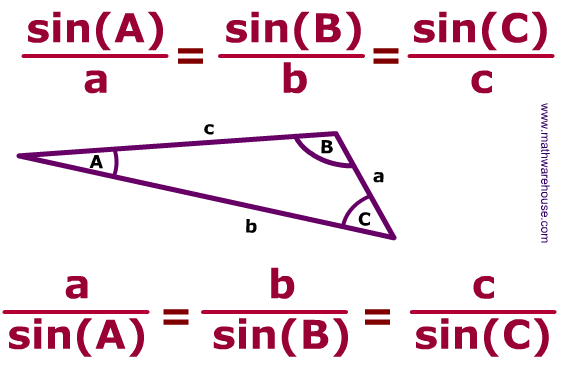Application of Sine Rule

• Sine rule can be used to find the unknown side or length of a triangle.
• Sine rule can also be used to find the unknown angle of a triangle.

To find the unknown side or length of a triangle, three essential parameters must be provided and these parameters are:

• The angle opposite to the unknown side or length of the triangle.
• The length of a side of the triangle (b).
• The angle opposite to the length of side (b) of the triangle.

Let’s take for example we have a triangle and we want to determine the unknown side of this triangle, when the opposite angle is given, another length of the side of the triangle and its opposite angle is also given. Like the image below.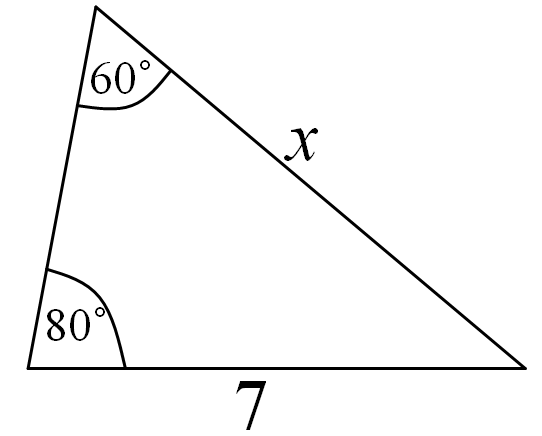From the image above, one can see that;
The angle opposite to the unknown length of the side of the triangle is 80°. (A)
The length of the side of the triangle given is 7. (b)
The angle opposite to the length of the side of the triangle given is 60° (B)
The unknown length of the side of the triangle is x. (a)

Applying the sine rule to find the value of x:

a / sin(A) = b / sin(B)

x / sin(80°) = 7 / sin(60°)
x / 0.9848 = 7 / 0.8660

Applying cross multiplication

x (0.8660) = 7 (0.9848)
x (0.8660) = 6.8936

Dividing both sides by 0.8660

x = 6.8936 / 0.8660
x = 7.96

Therefore, the unknown length of the side of the triangle, x is 7.96.

## How to Calculate and Solve for the Angle, Radius and Length of an Arc of a Circle | The Calculator Encyclopedia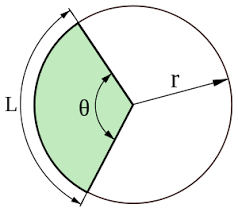The image above represents the length of an arc of a circle.

To compute the length of an arc of a circle, two essential parameters are needed and this parameters are radius of the circle (r) and angle of the circle (α).

The formula for calculating the length of an arc of a circle:

L = απr / 180

Where;
L = Length of an arc of the circle
α = Angle of the circle
r = radius of the circle

Let’s solve an example:
Find the length of an arc of a circle when the angle of the circle is 90° and the radius of the circle is 20 cm.

This implies that;
α = Angle of the circle = 90°
r = Radius of the circle = 20 cm

L = απr / 180
L = 90 x 3.142 x 20 / 180
L = 5655.6 / 180
L = 31.42

Therefore, the length of an arc of the circle is 31.42 cm.

Calculating the Angle of a Circle using the Radius of the Circle and Length of an Arc of the Circle.

α = 180L / πr

Where;
L = Length of an arc of the circle
r = Radius of the circle

Let’s solve an example;
Find the angle of the circle when the length of an arc of the circle is 60° and a radius of 140 cm.

This implies that;
L = Length of an arc of the circle = 60°
r = Radius of the circle = 140 cm

α = 180L / πr
α = 180 x 60 / 3.142 x 140
α = 10800 / 439.88
a = 24.55

Therefore, the angle of the circle is 24.55°.

## How to Calculate and Solve for the Perimeter or Circumference, Diameter, Radius and Angle of a Sector | The Calculator Encyclopedia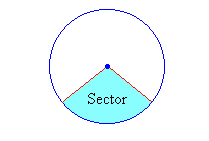The image above is a sector.

To compute the Perimeter or Circumference a sector, two essential parameters is needed and this parameters are the radius of the sector (r) and the angle of the sector (θ). You can also use the diameter of the sector (d).

The formula for calculating the Perimeter or Circumference of a sector:

P = 2r + θ / 360(2πr)

Where;

P = Perimeter or Circumference
r = Radius of the sector
θ = Angle of the Sector

Let’s solve an example:
Find the perimeter or circumference of a sector when the radius of the sector is 14 cm and the angle of the sector is 60°

This implies that;

r = Radius of the sector = 14 cm
θ = Angle of the sector = 60°

P = 2r + θ / 360(2πr)
P = 2 x 14 + 60 / 360(2 x 3.142 x 14)
P = 28 + 0.1667 (87.97)
P = 28 + 14.66
P = 42.66

Therefore, the perimeter or circumference of the sector is 42.66 cm.

Calculating the Perimeter or Circumference of a Sector using Diameter and Angle of the sector.

P = d + θ / 360(πd)

θ = Angle of the sector
d = Diameter of the sector

Let’s solve an example;
Find the perimeter or circumference of a sector when the diameter of the sector is 20 cm and the angle of the sector is 80°.

This implies that;

d = Diameter of the sector = 20 cm
θ = Angle of the sector = 80°

## How to Calculate and Solve for the Area, Radius, Diameter and Angle of a Sector | The Calculator Encyclopedia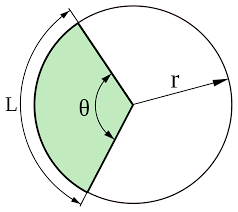The image above is a sector.

To compute the area of a sector, two essential parameters is needed and this parameters are the radius of the sector (r) and the angle of the sector (θ). You can also use the diameter of the sector (d).

The formula for calculating the area of a sector:

Area of a sector = (θ/360)[πr²]

Where;

A = Area of the Sector
r = Radius of the Sector
θ = Angle of the Sector

Let’s solve an example:
Find the area of a sector when the radius of the sector is 7 cm and the angle of the sector is 9°

This implies that;

r = Radius of the sector = 7 cm
θ = Angle of the sector = 9°

A = θ / 360 x πr2
A = (9/360)[π x 7²]
A = 0.025 x π x 49
A = 3.848

Therefore, the area of the sector is 3.848 cm2.

Calculating the Area of a Sector using Diameter and Angle of the sector.

The formula is A = θ / 360 x πd2 / 4

Where;

θ = Angle of the sector
d = Diameter of the sector

Let’s solve an example;
Find the Area of a sector when the diameter of the sector is 12 cm and the angle of the sector is 18°.

This implies that;

d = Diameter of the sector = 12 cm
θ = Angle of the sector = 18°

A = θ / 360 x πd2 / 4
A = 18 / 360 x 3.142 (144) / 4
A = 18 / 360 x 452.448 / 4
A= 18 / 360 x 113.112
A= 0.05 x 113.112
A = 5.656

Therefore, the area of the sector with diameter is 5.656 cm

How to Calculate Angle of a Sector when Area of the Sector and Radius of the Sector is Given

θ = 360 (A) / πr2

where;

r = Radius of a sector
A = Area of a sector

Let’s solve an example;
Given that the area of a sector is 15 cm2 and the radius of the sector is 5 cm. Find the angle of the sector?

This implies that;
A = Area of the sector = 15 cm2
r = Radius of the sector = 5 cm

θ = 360 (A) / πr2
θ = 360 (15) / 3.142 (5)2
θ = 5400 / 3.142 (25)
θ = 5400 / 78.55
θ = 68.746

Therefore, the angle of the sector is 68.746°.

How to Calculate Angle of a Sector when Area of the Sector and Diameter of the Sector is Given

θ = 1440 (A) / πd2

where;

d = Diameter of a sector
A = Area of a sector

Let’s solve an example;
Given that the area of a sector is 22 cm2 and the diameter of the sector is 10 cm. Find the angle of the sector?

This implies that;
A = Area of the sector = 22 cm2
d = Diameter of the sector = 10 cm

θ = 1440 (A) / πr2
θ = 1440 (22) / 3.142 (10)2
θ = 31680 / 3.142 (100)
θ = 31680 / 314.2
θ = 100.88

Therefore, the angle of the sector is 100.88°.

How to Calculate Diameter of a Sector when Area of the Sector and Angle of the Sector is Given

d = √1440 (A) / πθ

where;

θ = Angle of a sector
A = Area of a sector

Let’s solve an example;
Given that the area of a sector is 24 cm2 and the angle of the sector is 10°. Find the diameter of the sector?

This implies that;
A = Area of the sector = 24 cm2
θ = Angle of the sector = 10°

d = √1440 (A) / πθ
d = √1440 (24) / 3.142 x 10
d = √34560 / 31.42
d = √1099.936
d = 33.165

Therefore, the diameter of the sector is 33.165 cm.

How to Calculate Radius of a Sector when Area of the Sector and Angle of the Sector is Given

r = √360 (A) / πθ

where;

θ = Angle of a sector
A = Area of a sector